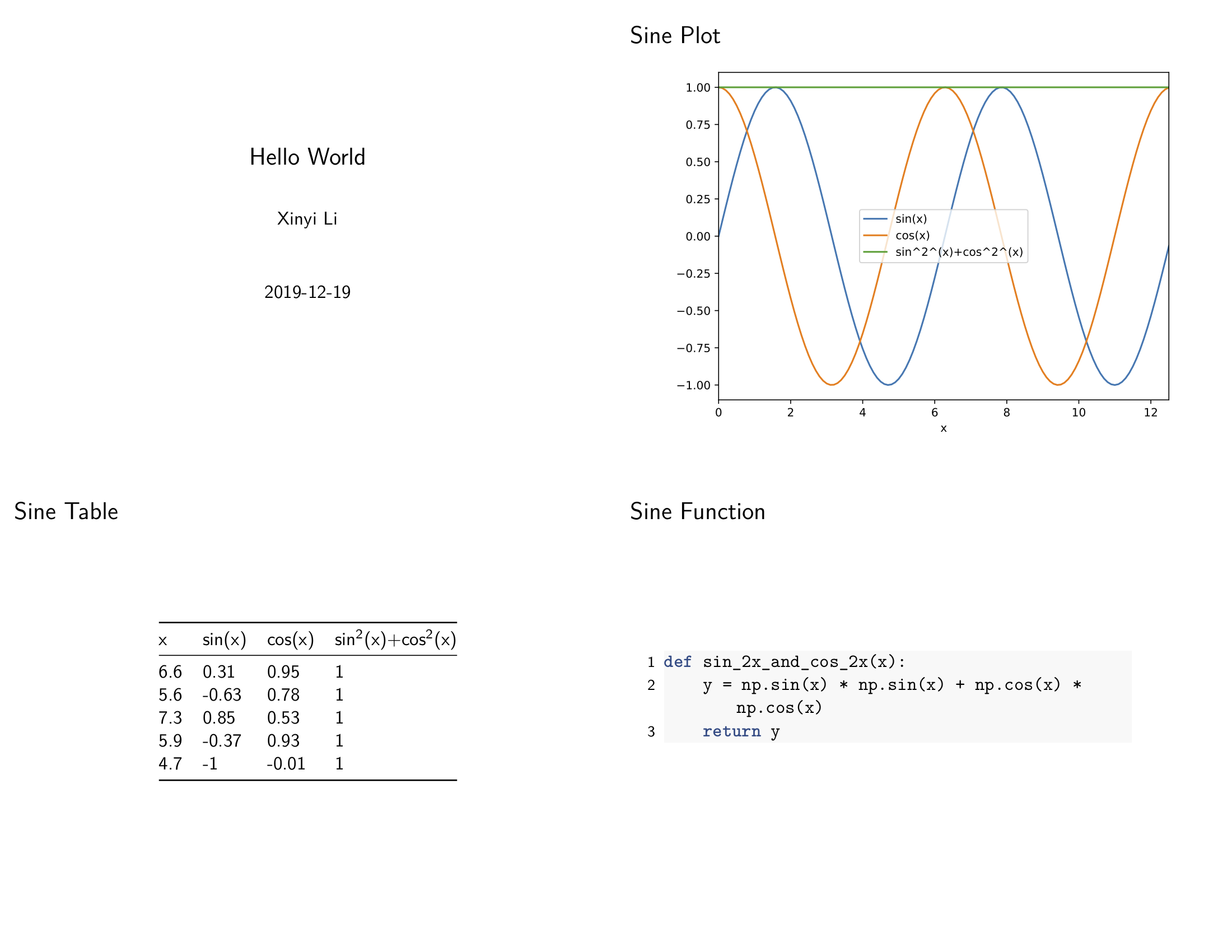# Quick Start¶

## Pre-requirements¶

Before installing `hkjournalist`, please make sure `pandoc` and `pdflatex` are already properly installed in the environment.

• `pandoc`: pandoc.org/installing.html
• `texlive`/`mactex`(for MacOS): [www.tug.org/texlive/ http://www.tug.org/mactex/](https://www.tug.org/texlive/ http://www.tug.org/mactex/)

## Install¶

```pip install hkjournalist
```

Write such a `md` file, use a pair of `{}` to wrap every variable which will be assigned specified value in your code. save it to `template.md`

```% Hello World
% Xinyi Li
% 2019-12-19

---

### sine plot

![]({sin_plot})

### sine table

{sin_table}

### sine function

```{{.python}}
{sin_func}
```
```

## Run a `Journalist()` in your code to fetch variables¶

First, you should define a `dict` to record mapping with variable names and their value

```from hkjournalist import Journalist

config = {}
```

Then, start your programming, and do not forget to assign value to corresponding variable names in `config`:

```def sin_2x_and_cos_2x(x):
y = np.sin(x) * np.sin(x) + np.cos(x) *  np.cos(x)
return y

x = np.arange(0, 4 * np.pi, 0.1)
y1 = np.sin(x)
y2 = np.cos(x)

df = pd.DataFrame({'x': x, 'sin(x)': y1, 'cos(x)': y2})
df['sin^2^(x)+cos^2^(x)'] = sin_2x_and_cos_2x(df['x']).values
df = df.set_index('x')

# plot sine curve as sin_plot
ax = df.plot()
plt.tight_layout()
config['sin_plot'] = ax

# random select 5 point (x,y) as sin_table
config['sin_table'] = df.sample(5)

# sin_2x_and_cons_2x as sin_func
config['sin_func'] = sin_2x_and_cos_2x
```

## Invite a journalist to make a big news report¶

Last but not least, attach 3 lines critical code below to have your `Journalist` make a report and save it to `big_news.pdf` (you can get all code above in demo and the output file big_news.pdf)

```# HK journalist runs faster than everyone! hear variable and make a report
reporter = Journalist(template_file='template.md')
reporter.hear(config)
reporter.report(output_file='big_news.pdf', beamer=True, overwrite=True)
```

Report slides display as below:## Final question: What will my variables on slides look like?¶

All variables pass to `Journalist` via `hear` will display as strings just like what their `__str__` method do.

Except for 4 types with special support:

• `pandas.DataFrame`: -> a 3-line table (`TeX` default style)
• `matplotlib.axes.SubplotBase` (known as base figure object `ax` in `matplotlib`): -> a figure print on report (with high quality and small size as `pdf`)
• `function`: -> its full definition
• `list(str)`: -> `len(list)` followed by a sentance with all words concatenated.